# 1604 and a Big Level One

### Today’s Puzzle:

1604 was my house number most of my childhood. Perhaps this number is making me feel a little more playful. I decided my next few puzzles would be based on a 1 to 14 multiplication table. I find that I personally use clues like 26, 39, 52, 65, 78, 104, 143, and 169 often in life. For example, why are there 52 playing cards in a deck of cards? To help you get more familiar with the 1 to14 multiplication table, we will start with this level one puzzle:Print the puzzles or type the solution in this excel file: 14 Factors 1604-1612.

### Factors of 1604:

• 1604 is a composite number.
• Prime factorization: 1604 = 2 × 2 × 401, which can be written 1604 = 2² × 401.
• 1604 has at least one exponent greater than 1 in its prime factorization so √1604 can be simplified. Taking the factor pair from the factor pair table below with the largest square number factor, we get √1604 = (√4)(√401) = 2√401.
• The exponents in the prime factorization are 2 and 1. Adding one to each exponent and multiplying we get (2 + 1)(1 + 1) = 3 × 2 = 6. Therefore 1604 has exactly 6 factors.
• The factors of 1604 are outlined with their factor pair partners in the graphic below.### More about the Number 1604:

1604 is the sum of two squares:
40² + 2² = 1604.

1604 is the hypotenuse of a Pythagorean triple:
160-1596-1604, calculated from 2(40)(2), 40² – 2², 40² + 2².
It is also 4 times (40-399-401).

# 1594 and Level 1

### Today’s Puzzle:

Write the numbers from 1 to 10 in the first column and the top row so that those numbers and the given clues function like a multiplication table.### Factors of 1594:

• 1594 is a composite number.
• Prime factorization: 1594 = 2 × 797.
• 1594 has no exponents greater than 1 in its prime factorization, so √1594 cannot be simplified.
• The exponents in the prime factorization are 1 and 1. Adding one to each exponent and multiplying we get (1 + 1)(1 + 1) = 2 × 2 = 4. Therefore 1594 has exactly 4 factors.
• The factors of 1594 are outlined with their factor pair partners in the graphic below.### More about the Number 1594:

1594 is the sum of two squares as well as the double of two squares:
37² + 15² = 1594.
2(26² + 11²) = 1594.

1594 is the hypotenuse of a Pythagorean triple:
1110-1144-1594, calculated from 2(37)(15), 37² – 15², 37² + 15².
It is also 2 times (555-572-797).

# 1586 and a Level One Puzzle

### Today’s Puzzle:

Write the number from 1 to 12 in both the first column and the top row of the puzzle so that the given clues are the products of the numbers you write.### Factors of 1586:

• 1586 is a composite number.
• Prime factorization: 1586 = 2 × 13 × 61.
• 1586 has no exponents greater than 1 in its prime factorization, so √1586 cannot be simplified.
• The exponents in the prime factorization are 1, 1, and 1. Adding one to each exponent and multiplying we get (1 + 1)(1 + 1)(1 + 1) = 2 × 2 × 2 = 8. Therefore 1586 has exactly 8 factors.
• The factors of 1586 are outlined with their factor pair partners in the graphic below.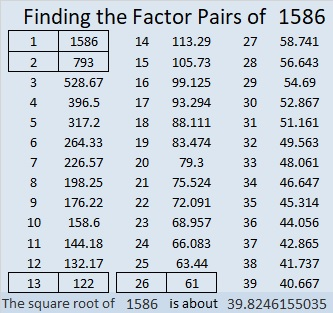### More about the Number 1586:

1586 is the sum of two squares in two different ways:
35² + 19² = 1586, and
31² + 25² = 1586.

1586 is the hypotenuse of FOUR Pythagorean triples:
286-1560-1586, which is 26 times (11-60-61),
336-1550-1586, calculated from 31² – 25², 2(31)(25), 31² + 25²,
610-1464-1586, which is (5-12-13) times 122, and
864-1330-1586, calculated from 31² – 25², 2(31)(25), 31² + 25².

# 1576 Why Do the Digits of Every Clue in This Puzzle Add up to Nine?

### Today’s Puzzle:

The digits of each clue in this puzzle add up to nine. Relatively few Find the Factors puzzles can make that claim. Why can this one make it?

Can you write all the numbers from 1 to 10 so that this puzzle will function like a multiplication table?### Factors of 1576:

1576 is divisible by a few powers of two, namely 1, 2, 4, and 8. Here is something for you to think about:

1576 is a whole number so it is divisible by 1.
6 is even so 1576 is divisible by 2.
7 is odd and 6 is not divisible by 4, so 1576 is divisible by 4.
5 is odd and 76 is not divisible by 8, so 1576 is divisible by 8.
1 is odd and 576 is divisible by 16, so 1576 is not divisible by 16.
(Each of those statements was also dependent on the statement before it.)

• 1576 is a composite number.
• Prime factorization: 1576 = 2 × 2 × 2 × 197, which can be written 1576 = 2³ × 197.
• 1576 has at least one exponent greater than 1 in its prime factorization so √1576 can be simplified. Taking the factor pair from the factor pair table below with the largest square number factor, we get √1576 = (√4)(√394) = 2√394.
• The exponents in the prime factorization are 3 and 1. Adding one to each exponent and multiplying we get (3 + 1)(1 + 1) = 4 × 2 = 8. Therefore 1576 has exactly 8 factors.
• The factors of 1576 are outlined with their factor pair partners in the graphic below.### More about the Number 1576:

1576 is the sum of two squares:
30² + 26² = 1576.

1576 is the hypotenuse of a Pythagorean triple:
224-1560-1576, calculated from 30² – 26², 2(30)(26), 30² + 26².
It is also 8 times (28-195-197).

1576 is also the difference of two squares in two different ways:
395² – 393² = 1576 and
199² – 195² = 1576.

# 1559 We Need a Little Christmas Now

### Today’s Puzzle:

2020 reminds me of another difficult year, 2001. In December of that year, Angela Lansbury sang We Need a Little Christmas Now with the Tabernacle Choir at Temple Square. I was able to watch the concert on television, and I remember the feeling the music brought me. What a wonderful gift music is! Yes, in 2020, we need a little Christmas now!

This level 2 puzzle brings a little Christmas now. Write the numbers from 1 to 12 in both the first column and the top row so that the puzzle functions as a type of multiplication table. I’m pretty sure you can figure it out!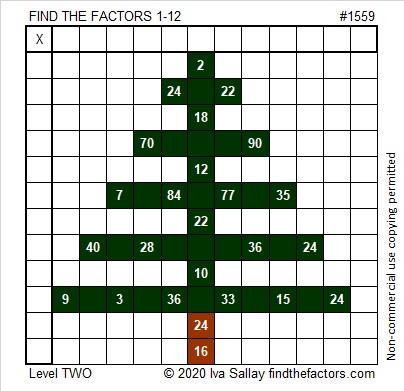### Factors of 1559:

• 1559 is a prime number.
• Prime factorization: 1559 is prime.
• 1559 has no exponents greater than 1 in its prime factorization, so √1559 cannot be simplified.
• The exponent in the prime factorization is 1. Adding one to that exponent we get (1 + 1) = 2. Therefore 1559 has exactly 2 factors.
• The factors of 1559 are outlined with their factor pair partners in the graphic below.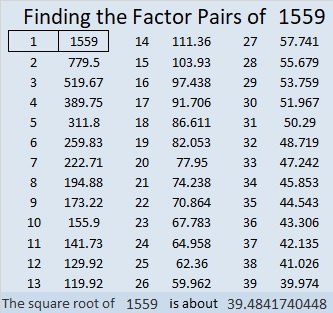How do we know that 1559 is a prime number? If 1559 were not a prime number, then it would be divisible by at least one prime number less than or equal to √1559. Since 1559 cannot be divided evenly by 2, 3, 5, 7, 11, 13, 17, 19, 23, 29, 31, or 37, we know that 1559 is a prime number.

### More about the number 1559:

1559 is the sum of two consecutive numbers:
780 + 779 = 1559.

1559 is also the difference of two consecutive square numbers:
780² – 779² = 1559.

(Yes, I know, any odd whole number can make a similar claim.)

# 1558 The Reason for the Season

### Today’s Puzzle:

What is the reason we have the Christmas season? The answer to that question actually begins with a cross…Write the numbers from 1 to 12 in both the first column and the top row so that the given clues are the products of those numbers.

### Factors of 1558:

• 1558 is a composite number.
• Prime factorization: 1558 = 2 × 19 × 41.
• 1558 has no exponents greater than 1 in its prime factorization, so √1558 cannot be simplified.
• The exponents in the prime factorization are 1, 1, and 1. Adding one to each exponent and multiplying we get (1 + 1)(1 + 1)(1 + 1) = 2 × 2 × 2 = 8. Therefore 1558 has exactly 8 factors.
• The factors of 1558 are outlined with their factor pair partners in the graphic below.### Another Fact about the Number 1558:

1558 is the hypotenuse of a Pythagorean triple:
342-1520-1558, which is 38 times (9-40-41).

# 1546 Celebrating My 7th Blogiversary

### Today’s Puzzle:

Seven years ago today at 2:11 in the morning, I published my first blog post. It featured a Find the Factors 1 – 10 puzzle with only perfect square clues. In honor of that first post, I publish this puzzle, also with only perfect square clues. This one looks like an exclamation point because I have enjoyed creating puzzles and writing these posts for you so much these seven years!How do you solve the puzzle? Use logic to write the numbers from 1 to 10 in both the first column and the top row so that the puzzle functions like a multiplication table.

### Factors of 1546:

• 1546 is a composite number.
• Prime factorization: 1546 = 2 × 773.
• 1546 has no exponents greater than 1 in its prime factorization, so √1546 cannot be simplified.
• The exponents in the prime factorization are 1 and 1. Adding one to each exponent and multiplying we get (1 + 1)(1 + 1) = 2 × 2 = 4. Therefore 1546 has exactly 4 factors.
• The factors of 1546 are outlined with their factor pair partners in the graphic below.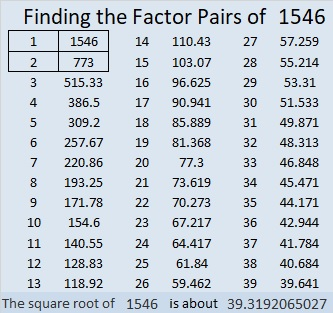### More about the Number 1546:

1546 is the sum of two squares:
39² + 5² = 1546.

1546 is the hypotenuse of a Pythagorean triple:
390-1496-1546, calculated from 2(39)(5), 39² – 5², 39² + 5².
It is also 2 times (195-748-773)

# 1542 Fourth Letter of the Message

### Today’s Puzzle:

This level one puzzle is the easiest of all the puzzles in my message to you. It might also be the letter that helps you know exactly what the message says.### Factors of 1542:

• 1542 is a composite number.
• Prime factorization: 1542 = 2 × 3 × 257.
• 1542 has no exponents greater than 1 in its prime factorization, so √1542 cannot be simplified.
• The exponents in the prime factorization are 1, 1, and 1. Adding one to each exponent and multiplying we get (1 + 1)(1 + 1)(1 + 1) = 2 × 2 × 2 = 8. Therefore 1542 has exactly 8 factors.
• The factors of 1542 are outlined with their factor pair partners in the graphic below.### Another Fact about the Number 1542

1542 = 1¹ + 5¹ + 4⁵ + 2 so it is a digitally powerful number.

1542 is the hypotenuse of a Pythagorean triple:
192-1530-1542, which is 6 times (32-255-257).

# 1526 Grave Marker

### Today’s Puzzle:

It’s almost Halloween! This is my favorite kind of grave marker, one that is really just a Find the Factors puzzle in disguise. It’s only a level one, so it isn’t very tricky. I hope you find it a real treat!Here’s the same puzzle but requiring less ink to print:Neighbors have decorated part of their yard to look like a mini graveyard for Halloween. I think my grave marker would fit right in!### Factors of 1526:

• 1526 is a composite number.
• Prime factorization: 1526 = 2 × 7 × 109.
• 1526 has no exponents greater than 1 in its prime factorization, so √1526 cannot be simplified.
• The exponents in the prime factorization are 1, 1, and 1. Adding one to each exponent and multiplying we get (1 + 1)(1 + 1)(1 + 1) = 2 × 2 × 2 = 8. Therefore 1526 has exactly 8 factors.
• The factors of 1526 are outlined with their factor pair partners in the graphic below.### Another Fact about the Number 1526:

1526 is the hypotenuse of a Pythagorean triple:
840-1274-1526 which is 14 times (60-91-109)

# 1511 and Level 1

### Today’s Puzzle:

Do you know what number is the common factor of all the clues in this puzzle? If you do, then you are well on your way to solving this level one puzzle!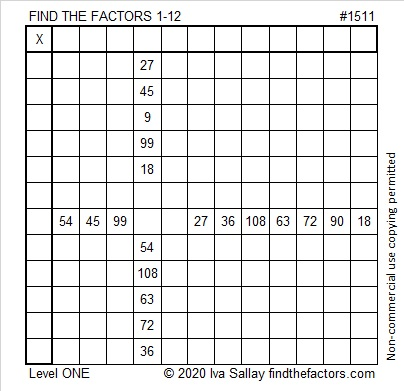### Factors of 1511:

• 1511 is a prime number.
• Prime factorization: 1511 is prime.
• 1511 has no exponents greater than 1 in its prime factorization, so √1511 cannot be simplified.
• The exponent in the prime factorization is 1. Adding one to that exponent we get (1 + 1) = 2. Therefore 1511 has exactly 2 factors.
• The factors of 1511 are outlined with their factor pair partners in the graphic below.How do we know that 1511 is a prime number? If 1511 were not a prime number, then it would be divisible by at least one prime number less than or equal to √1511. Since 1511 cannot be divided evenly by 2, 3, 5, 7, 11, 13, 17, 19, 23, 29, 31, or 37, we know that 1511 is a prime number.

### One More Fact about the Number 1511:

1511 is equidistant from the two closest primes:
The previous prime was 1511 – 12 = 1499.
The next prime will be 1511 + 12 = 1523.